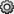Documentation

## Display Format for Numeric Values

By default, MATLAB® uses a 5-digit short format to display numbers. For example,

`x = 4/3`
```x = 1.3333```

You can change the display in the Command Window or Editor using the `format` function.

```format long x```
```x = 1.333333333333333```

Using the `format` function only sets the format for the current MATLAB session. To set the format for subsequent sessions, clickon the Home tab in the Environment section. Select MATLAB > Command Window, and then choose a Numeric format option.

The following table summarizes the numeric output format options.

`Style`

Result

Example

`short (default)`

Short, fixed-decimal format with 4 digits after the decimal point.

`3.1416`

`long`

Long, fixed-decimal format with 15 digits after the decimal point for `double` values, and 7 digits after the decimal point for `single` values.

`3.141592653589793`

`shortE`

Short scientific notation with 4 digits after the decimal point.

`3.1416e+00`

`longE`

Long scientific notation with 15 digits after the decimal point for `double` values, and 7 digits after the decimal point for `single` values.

`3.141592653589793e+00`

`shortG`

Short, fixed-decimal format or scientific notation, whichever is more compact, with a total of 5 digits.

`3.1416`

`longG`

Long, fixed-decimal format or scientific notation, whichever is more compact, with a total of 15 digits for `double` values, and 7 digits for `single` values.

`3.14159265358979`

`shortEng`

Short engineering notation (exponent is a multiple of 3) with 4 digits after the decimal point.

`3.1416e+000`

`longEng`

Long engineering notation (exponent is a multiple of 3) with 15 significant digits.

`3.14159265358979e+000`

`+`

Positive/Negative format with `+`, `-`, and blank characters displayed for positive, negative, and zero elements.

`+`

`bank`

Currency format with 2 digits after the decimal point.

`3.14`

`hex`

Hexadecimal representation of a binary double-precision number.

`400921fb54442d18`

`rat`

Ratio of small integers.

`355/113`

The display format only affects how numbers are displayed, not how they are stored in MATLAB.